## General Features of Helium States

We can use the hydrogenic states to begin to understand Helium. The Hamiltonian has the same terms as Hydrogen but has a large perturbation due to the repulsion between the two electrons.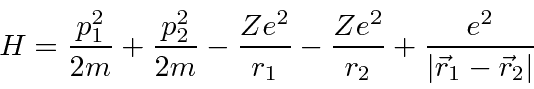We can write this in terms of the () Hydrogen Hamiltonian for each electron plus a perturbation,where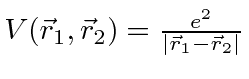. Note that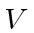is about the same size as the the rest of the Hamiltonian so first order perturbation theory is unlikely to be accurate.

For our zeroth order energy eigenstates, we will use product states of Hydrogen wavefunctions.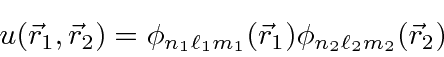These are not eigenfunctions of H because of, the electron coulomb repulsion term. Ignoring, the problem separates into the energy for electron 1 and the energy for electron 2 and we can solve the problem exactly.We can write these zeroth order energies in terms of the principal quantum numbers of the two electrons,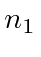and. Recalling that there is a factor ofin these energies compared to hydrogen, we get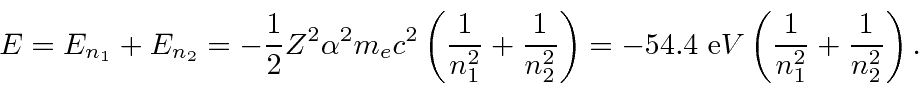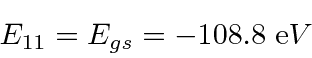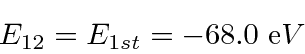Note thatis above ionization energy, so the state can decay rapidly by ejecting an electron.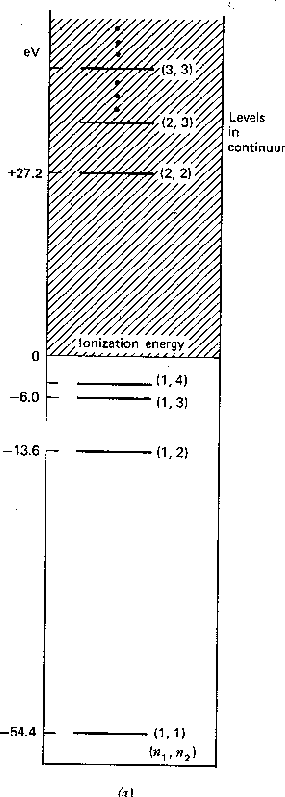Now let's look at the (anti) symmetry of the states of two identical electrons. For the ground state, the spatial state is symmetric, so the spin state must be antisymmetric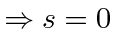.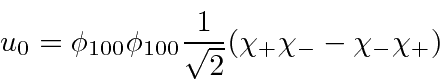For excited states, we can make either symmetric or antisymmetric space states.The first state isor spin singlet. The second state isor spin triplet and has threestates. Only the +1 state is shown. Because the large correction due to electron repulsion is much larger for symmetric space states, the spin of the state determines the energy.

We label the states according to the spin quantum numbers, singlet or triplet. We will treat V as a perturbation. It is very large, so first order perturbation theory will be quite inaccurate.

Jim Branson 2013-04-22